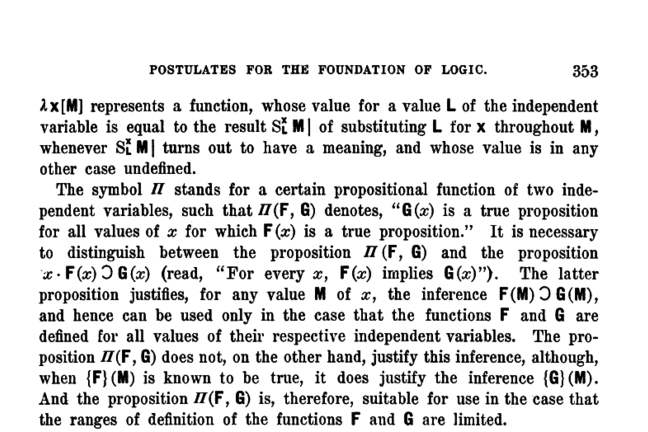6.5

## University of GlasgowExcerpt from "Postulates for the foundation of Logic" by A. Church, 1932

# Introduction to the Lambda calculus

### Introduction to the Lambda Calculus

• The lambda calculus was developed in the 1930s by Alonzo Church (1903–1995), one of the leading developers of mathematical logic.

• The lambda calculus was an attempt to formalise functions as a means of computing.

### Significance to computability theory

• A major (really the major) breakthrough in computability theory was the proof that the lambda calculus and the Turing machine have exactly the same computational power.

• This led to Church’s thesis — that the set of functions that are effectively computable are exactly the set computable by the Turing machine or the lambda calculus.

• The thesis was strengthened when several other mathematical computing systems (Post Correspondence Problem, and others) were also proved equivalent to lambda calculus.

• The point is that the set of effectively computable functions seems to be a fundamental reality, not just a quirk of how the {Turing machine, lambda calculus} was defined.

### Significance to programming languages

• The lambda calculus has turned out to capture two aspects of a function:

• A mathematical object (set or ordered pairs from domain and range), and

• An abstract black box machine that takes an input and produces an output.

• The lambda calculus is fundamental to denotational semantics, the mathematical theory of what computer programs mean.

• Functional programming languages were developed with the explicit goal of turning lambda calculus into a practical programming language.

• The ghc Haskell compiler operates by (1) desugaring the source program, (2) transforming the program into a version of lambda calculus called System F, and (3) translating the System F to machine language using graph reduction.

### Abstract syntax of lambda calculus

• We will work with the basic lambda calculus “enriched” with some constants and primitive functions (strictly speaking, that is not necessary).

• The language has constants, variables, applications, and functions.


exp
= const
| var
| exp exp
| \ var -> exp


### Variables

• Each occurrence of a variable in an expression is either bound or free

• In $\backslash x \rightarrow x+1$, the occurrence of $x$ in $x+1$ is bound by the $\backslash x$.

• In $y*3$, the occurrence or $y$ is free. It must be defined somewhere else, perhaps as a global definition.

• In general, an occurrence of a variable is bound if there is some enclosing lambda expression that binds it; if there is no lambda binding, then the occurrence if free.

We need to be careful: the first occurrence of $a$ is free but the second occurrence is bound.

       a + (\ a -> 2^a) 3  -- >   a + 2^3


Being free or bound is a property of an occurrence of a variable, not of the variable itself!

### Conversion rules

• Computing in the lambda calculus is performed using three conversion rules.

• The conversion rules allow you to replace an expression by another (“equal”) one.

• Some conversions simplify an expression; these are called reductions.

### Alpha conversion

• Alpha conversion lets you change the name of a function parameter consistently.

• But you can’t change free variables with alpha conversion!

• The detailed definition of alpha conversion is a bit tricky, because you have to be careful to be consistent and avoid “name capture”. We won’t worry about the details right now.

(\x -> x+1) 3
(\y -> y+1) 3


### Beta conversion

• Beta conversion is the “workhorse” of lambda calculus: it defines how functions work.

• To apply a lambda expression an argument, you take the body of the function, and replace each bound occurrence of the variable with the argument.

    (\x -> exp1) exp2


is evaluated as $exp1[exp2/x]$

Example:

    (\x -> 2*x + g x) 42


is evaluated as $2*42 + g \;42$

### Eta conversion

• Eta conversion says that a function is equivalent to a lambda expression that takes an argument and applies the function to the argument.
(\x -> f x)


is equivalent to $f$

Example (recall that $(*3)$ is a function that multiplies its argument by 3)

(\x -> (*3) x)


is equivalent to $(*3)$

Try applying both of these to 50:

(\x -> (*3) x) 50


is the same as $(*3) \;50$

### Removing a common trailing argument

There is a common usage of Eta conversion. Suppose we have a definition like this:

f x y = g y


This can be rewritten as follows:

f = \x -> (\y -> g y)
f = \x -> g = f x = g


Thus the following two definitions are equivalent:

    f x y = g y
f x = g


In effect, since the last argument on both sides of the equation is the same ($y$), it can be “factored out”.